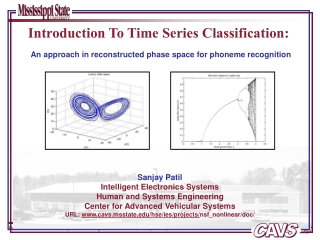DownloadDownload PresentationIntroduction To Time Series Classification:

# Introduction To Time Series Classification:

Download Presentation## Introduction To Time Series Classification:

- - - - - - - - - - - - - - - - - - - - - - - - - - - E N D - - - - - - - - - - - - - - - - - - - - - - - - - - -
##### Presentation Transcript

1. Introduction To Time Series Classification: An approach in reconstructed phase space for phoneme recognition Sanjay Patil Intelligent Electronics Systems Human and Systems Engineering Center for Advanced Vehicular Systems URL: www.cavs.msstate.edu/hse/ies/projects/nsf_nonlinear/doc/

2. Abstract • Present nonlinear classifiers: • clustering and similarity measurement techniques, eg. NN, SVM. • Existing time-domain approaches: • a priori learned underlying pattern of template base. • Frequency-based techniques: • spectral patterns based on first and second order characteristics of the system. • Current work (as described in the paper): • modeling of signals in the reconstructed phase space.

3. Slightly different notations than usually used by other researchers. • Motivation (why did I read it?) An attempt to find an approach to model the speech signal using nonlinear modeling technique. • Takens and Sauer – new signal classification algorithm. • Time series of observations sampled from a single state variable of a system • Reconstructed space equivalent to the original system

4. The Approach • Two methods to tackle the issue: • Build global vector reconstructions and differentiate signals in a coefficient space. [Kadtke, 1995] • Build GMMs of signal trajectory densities in an RPS and differentiate between signals using Bayesian classifiers. [Authors, 2004] • The steps (Algorithm): • Data Analysis – normalizing the signals, estimating the time lag and dimension of the RPS. • Learning GMMs for each signal class – deciding the number of Gaussian mixtures, parameters learning by Expectation-Maximization (EM) algorithm. • Classification – going through the above steps for the SUT (signal under test), using Bayesian maximum likelihood classifiers

5. Algorithm in details and Issues • Data Analysis – • normalizing the signals • Each signal is normalized to zero mean and unit standard deviation. • estimating the time lag  • Using first minimum of the automutual information function. • Overall time lag  is the mode of the histogram of the first minima for all signals. • estimating dimension d of the RPS • Using global false nearest-neighbor technique. • Overall RPS dimension is the mean plus two standard deviations of the distribution of individual signal RPS dimensions. • How do you normalize the signal to zero mean and unit standard deviation? • What is automutual information function? • How do you implement the global false nearest-neighbor technique?

6. Algorithm in details and Issues • 2. Gaussian Mixture Models – • Insert all the signals for a particular class into the RPS for a particular d and  selected in previous step, • GMM: • Where, M = # of mixtures, • N(x;, ) = normal distribution with mean  and covariance matrix  • W = mixture weight with the constraint • GMMs estimated using Expectation-Maximization (EM) algorithm. • How is EM algorithm implemented? • Classification accuracy depends on M, So how to determine the value of M? • What is value of M determined from the underlying distribution of the RPS density?

7. Algorithm in details and Issues • 3. Classification – • Maximum Likelihood estimates from previous step are: • Where, mean , covariance matrix , mixture weight W • Using Bayesian maximum likelihood classifiers: • Compute the conditional likelihoods of the signal under each learned model • Select the model with highest likelihood. • How are the conditional likelihoods computed?

8. Experiment details and Issues • TIMIT speech corpus: • 417 phonemes for speaker MJDE0. • 6 spoken only once, 47 classes in total (out of the standard 48 classes) • Sampling frequency 16KHz, Signal length – 227 to 5,201 samples • Phoneme boundaries and class labels determined by a group of experts • 25 iterations of EM algorithm are used. • Classification accuracy is around 50% (50% for 16GMMs, @48% for 32GMMs) [reason – due to insufficient training data] • Approach is compared with time delay NN with nonlinear one step predictor and minimum prediction error classifier. • Details on how the testing is done is missing. • How is insufficient training data causing reduction in accuracy for increase in GM mixtures?

9. References • R. Povinelli, M. Johnson, A. Lindgren, and J. Ye, “Time Series Classification using Gaussian Mixture Models of Reconstructed Phase Spaces,” IEEE Transactions on Knowledge and Data Engineering, Vol 16, no 6, June 2004, pp. 770-783. (the referred paper) • F. Takens, “Detecting Strange Attractors in Turbulence,” Proceedings Dynamical Systems and Turbulence, 1980, pp 366-381. (background theory) • T. Sauer, J. Yorke, and M. Casdagli, “Embedology,” JournalStatistical Physics, vol 65, 1991, pp 579-616. (background theory) • A. Petry, D. Augusto, and C. Barone, “Speaker Identification using Nonlinear Dynamical Features,” Choas, Solitions, and Fractals, vol 13, 2002, pp 221-231. (speech related dynamical system) • H. Boshoff, and M. Grotepass, “The fractal dimension of fricative Speech Sounds,” Proceddings South African Symposium Communication and Signal Processing, 1991, pp 12-61. (speech related dynamical system) • D. Sciamarella and G. Mindlin, “Topological Structure of Chaotic Flows from Human Speech Chaotic Data,” Physical Review Letters, vol. 82, 1999, pp 1450. (speech related dynamical system) • T. Moon, “The Expectation-Maximization algorithm,” IEEE Signal Processing Magazine, 1996, pp 47-59. (expectation-maximization algorithm details) • Q. Ding, Z. Zhuang, L. Zhu, and Q. Zhang, “Application of the Chaos, Fractal, and Wavelet Theories to the Feature Extraction of Passive Acoustic Signal,” Acta Acustica, vol 24, 1999, pp 197-203. (frequency based speech dynamical system analysis) • J. Garofolo, L. Lamel, W. Fisher, J. Fiscus, D. Pallet, N. Dahlgren, and V. Zue, “TIMIT Acoustic-Phonetic Continuous Speech Corpus,” Linguistic Data Consortium, 1993. (speech data set used for experiments)